International
Tables for
Crystallography
Volume F
Crystallography of biological macromolecules
Edited by E. Arnold, D. M. Himmel and M. G. Rossmann

International Tables for Crystallography (2012). Vol. F, ch. 2.2, pp. 65-68

## Section 2.2.2. Quality indicators for diffraction data

H. M. Einspahra* and M. S. Weissb

aPO Box 6483, Lawrenceville, NJ 08648–0483, United States, and bHelmholtz-Zentrum Berlin für Materialien und Energie, Macromolecular Crystallography (HZB-MX), Albert-Einstein-Str. 15, D-12489 Berlin, Germany
Correspondence e-mail:  hmeinspahr@yahoo.com

### 2.2.2. Quality indicators for diffraction data

| top | pdf |

Once useful crystalline samples have been obtained, the collection of X-ray diffraction data is the next (and the last) experimental step in a structure determination. Although the greatest care may be taken to collect data of as high quality as possible, there remain circumstances and influences that limit the quality of the data. Over time, many indicators have been defined to describe various aspects of diffraction data quality. The most important ones are discussed here.

Nominal resolution, dmin. The resolution of a diffraction data set describes the extent of measurable data and is calculated by Bragg's law [equation (2.2.2.1)] based on the maximum Bragg angle 2θ included in the data set for a given data-collection wavelength λ. As discussed above, the nominal resolution is a limit set by the experimenters and is well known to be prone to subjective judgment. A number of suggestions have been made to reduce the subjectivity associated with this limit. One defines the limit as the resolution within which the intensities of a fraction of the unique reflections, for example 70%, are above a threshold, for example zero or three times their standard uncertainties. Another suggested limiting criterion, discussed further below, recommends that the nominal resolution be set as the midpoint of the resolution range of the shell at which the mean signal-to-noise ratio falls below 2.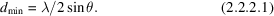True resolution, dtrue. The true resolution of a diffraction data set is defined as the minimum distance between two objects in a crystal that permits their images in the resultant electron-density map to be resolved. Often, dtrue is approximated as dmin.

To illustrate this crucial distance, represent two equivalent atoms by equal overlapping Gaussians. One might then consider that the distance between them that just permits distinguishing them as individual atoms might be the distance at which the electron-density value at the midpoint between the atoms drops to a value just below that at the positions of the atoms. For a normal distribution, this distance is 2σ, twice the standard deviation of the distribution.

Another perspective is provided by the realization that, when a Fourier synthesis is terminated at a resolution cutoff dmin, successive spheres of negative and positive density of decreasing amplitude surround the maxima of positive density at atomic positions in that synthesis. It has been shown that the distance from the centre of such a maximum to the first zero is 0.715dmin (James, 1948), which is a useful estimate of the limiting distance between distinguishable features in the electron-density map or dtrue. Similar estimates are used in other areas, notably for defining resolution in astronomy. A more recent re-evaluation suggests that a limit of 0.917dmin is a better value, especially when the effects of form factors and atomic displacement parameters are considered (Stenkamp & Jensen, 1984). Add to that the effects of errors in experimental amplitudes and derived phases, and the approximation of dtrue as dmin seems quite reasonable.

Optical resolution, dopt. The optical resolution dopt is calculated from the standard deviation of a Gaussian fitted to the origin peak of the Patterson function (σPatt) of the diffraction data set and the standard deviation of another Gaussian fitted to the origin peak of the spherical interference function (σsph). This definition is based on Vaguine et al. (1999) and is implemented in the program SFCHECK. The optical resolution is intended to account for uncertainties in the data, atomic displacement factors, effects of crystal quality and series-termination effects by means of a propagation-of-error-like approach (Blundell & Johnson, 1976; Vaguine et al., 1999). It has been suggested that dopt is a better approximation of dtrue than dmin (Weiss, 2001).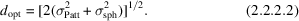Completeness, C. The completeness C of a diffraction data set is defined as the fraction of the unique reflections in a given space group to a given nominal resolution dmin that have been measured at least once during data collection. C may be given assuming that Friedel symmetry is either applied or not. In the latter case, C is also referred to as anomalous completeness. In the program SCALA (Evans, 2006), the anomalous completeness is defined based on acentric reflections only.

Effective resolution, deff. Since any missing reflection of a data set leads to a deterioration of the model parameters (Hirshfeld & Rabinovich, 1973; Arnberg et al., 1979), an effective resolution may be defined based on the nominal resolution dmin and the cube root of the completeness C of the data set.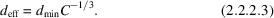Multiplicity (or redundancy), N. The multiplicity or redundancy N of a diffraction data set defines on average how many times a reflection hkl has been observed during the data-collection experiment including symmetry mates and replicate measurements. N may be given assuming that Friedel symmetry is either applied or not.

Merging R factor, Rmerge. The merging R factor of a diffraction data set describes the spread of the individual intensity measurements Ii of a reflection hkl around the mean intensity <I(hkl)> of this reflection. Sometimes Rmerge is also referred to as R factor (observed) (in the program XDS; Kabsch, 1988, 1993, 2010; Chapter 11.6), as Rsym or as Rlinear (in the program SCALEPACK; Otwinowski & Minor, 1997). In fractional form, this is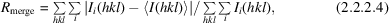where <I(hkl)> is the mean of the several individual measurements Ii(hkl) of the intensity of reflection hkl. The sums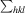and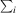run over all observed unique reflections hkl and over all individual observations i of a given reflection hkl. It should be noted that alternative definitions of Rmerge exist. In one, Ii(hkl) in the denominator is replaced by <I(hkl)>, thereby producing an expression that is formally equivalent to the one above. In another, Ii(hkl) in the denominator is replaced by |Ii(hkl)| with the suggestion that the denominator is thereby prevented from becoming negative or zero, even in the case of many negative-intensity observations. One should note, however, the counterintuitive side effect: artificial damping of Rmerge values, that is, reducing expected higher Rmerge values of data sets with more weak reflections.

The usefulness of Rmerge as a quality indicator for diffraction data is limited because it is dependent on the multiplicity of a data set (Diederichs & Karplus, 1997a,b; Weiss & Hilgenfeld, 1997; Weiss, 2001). The higher the multiplicity of a data set, the higher its Rmerge will be, although, based on statistics, the better determined the averaged intensity values should be. Despite these shortcomings, Rmerge is still widely used today.

Redundancy-independent merging R factor, Rr.i.m. or Rmeas. The redundancy-independent merging R factor Rr.i.m. or Rmeas describes the precision of the individual intensity measurements Ii, independent of how often a given reflection has been measured. Because of its independence of the redundancy (hence its name), it has been proposed that Rr.i.m. or Rmeas should be used as a substitute for the conventional Rmerge (Diederichs & Karplus, 1997a,b; Weiss & Hilgenfeld, 1997; Weiss, 2001). In fractional form, this is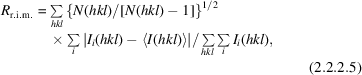where <I(hkl)> is the mean of the N(hkl) individual measurements Ii(hkl) of the intensity of reflection hkl. As for Rmerge, the sumsandrun over all observed unique reflections hkl and over all individual observations i of a given reflection hkl.

Precision-indicating merging R factor, Rp.i.m.. The precision-indicating merging R factor Rp.i.m. describes the precision of the averaged intensity measurements <I(hkl)> (Weiss, 2001). In fractional form, this is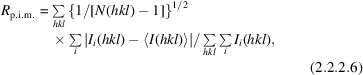where <I(hkl)> is the mean of the N(hkl) individual measurements Ii(hkl) of the intensity of reflection hkl. As with Rmerge and Rr.i.m. or Rmeas, the sumsandrun over all observed unique reflections hkl and over all individual observations i of a given reflection hkl.

R factor of merged intensities or amplitudes, Rmrgd-I and Rmrgd-F. An alternative precision-indicating merging R factor, called Rmrgd, is defined as the R factor between two or more data sets or between two subsets of a data set created by randomly apportioning the individual intensity measurements between the two subsets (Diederichs & Karplus, 1997a,b). Rmrgd can be calculated for intensities (Rmrgd-I) or structure-factor amplitudes (Rmrgd-F). The latter quantity was suggested to present a lower limit for the crystallographic R factor of a model against the observed data (Diederichs & Karplus, 1997a,b). In fractional form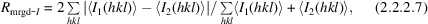where <I1(hkl)> and <I2(hkl)> are the mean intensity values for the individual observations of the reflections hkl, which have been partitioned into the two subsets 1 and 2. The sumsrun over all observed unique reflections. Rmrgd-I is related to Rp.i.m. by a constant factor (Rmrgd-I = 21/2Rp.i.m.).

Rmrgd-F is defined analogously to Rmrgd-I (Diederichs & Karplus, 1997a,b). In the equation, only the intensities are replaced by structure-factor amplitudes.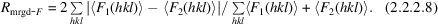In order to cope with negative-intensity observations, pseudo-amplitudes had to be introduced just for the purpose of calculating Rmrgd-F (F = I1/2 if I ≥ 0 and F = −|I|1/2 if I < 0).

Note. The approach of comparing randomly partitioned subsets of a given data set is used for a variety of quality indicators. While there is potential for variation in these indicators from one partitioning of the data set to another, an average of several random partitionings should be expected to give a useful estimate. There is also potential for subjectivity, but the principal value of these indicators is to assist the experimenter in proper analysis and they are less often applied to compare experiments from different laboratories and are seldom published.

Pooled coefficient of variation, PCV. The pooled coefficient of variation PCV is the ratio of the sum of the standard deviations to the sum of the reflection intensities (Diederichs & Karplus, 1997a,b). PCV is related to Rmeas or Rr.i.m. by the factor (π/2)1/2. In fractional form, this is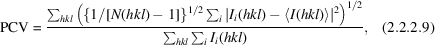where <I(hkl)> is the mean of the N(hkl) individual measurements Ii(hkl) of the intensity of reflection hkl.

Mean signal-to-noise ratio, <I/σ(I)>. The signal-to-noise ratio Ii/σ(Ii) of an individual intensity measurement describes the statistical significance of a measured intensity. As a measure of the overall quality of a data set, the mean signal-to-noise ratio for all reflections is useful as an indication of the robustness of the data, that is, the average intensity as a multiple of the standard uncertainty. In addition, as mentioned above, the mean signal-to-noise ratio for all reflections within the outer resolution shell can be used to define the nominal resolution of a data set. For the data set as a whole or for a resolution shell of that data set, the mean signal-to-noise ratio, <I/σ(I)>, is the sum of the signal-to-noise ratios of all individual reflections hkl within resolution limits divided by the number of individual reflections hkl within those resolution limits.

In principle there are two ways to define a mean signal-to-noise ratio of a data set (or a given resolution shell). The two ways yield different quantities, although, unfortunately, they are both called the mean signal-to-noise ratio. They differ in the manner in which mean signal-to-noise ratios of individual reflections hkl are calculated.

 (i) /σ[I(hkl)]. The mean signal-to-noise ratio of individual reflections hkl may be calculated as the ratio of the mean intensity and the r.m.s. scatter of Ii(hkl) about that mean. This is a measure of the average significance of individual observations, but it does not take into account the multiplicity or redundancy of the measurements.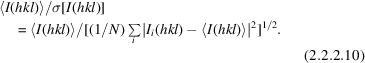In the program SCALA (Evans, 2006), this value is reported as I/sigma. (ii) . is the average over all observations of the reflection hkl, and is sometimes weighted. σ is the propagation-of-error combination of standard uncertainties assigned at data processing for the individual measurements Ii(hkl), that is, a modification of equation (2.2.2.10)in which the term |Ii(hkl) − | in the denominator is replaced by σi(hkl), the experimental standard uncertainty for the measurement Ii(hkl).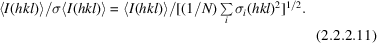An error model1 is often applied in the denominator here to scale to the r.m.s. scatter in (i) above. In the program SCALA (Evans, 2006), this value is reported as Mn(I)/sd.

Both methods of defining the mean signal-to-noise ratio for the reflection hkl have merit. As suggested for individual intensities in Section 2.2.1, perhaps the best approach would be to calculate weighted averages and weighted standard uncertainties of the I(hkl) where weights are the experimental standard uncertainties σi(hkl) for individual measurements Ii(hkl).

Highest possible signal-to-noise ratio, I/σ(I)asymptotic. A relatively recent addition to the collection of diffraction-data quality indicators is the highest possible signal-to-noise ratio of a data set I/σ(I)asymptotic or ISa (Diederichs, 2010). ISa is calculated from the parameters of the error model used for inflating the standard deviations of the reflections with an intensity-dependent term.1 Since ISa is practically independent of counting statistics, it was suggested to be a good measure of instrument errors manifesting themselves in the data set, provided the crystal is close to ideal and radiation damage is negligible. Data sets with ISa values of 25 or greater are considered to be very good and amenable to straightforward structure determination, while data sets exhibiting ISa values of 15 or less are considered marginal at best. The calculation of ISa is implemented in XDS versions of December 2009 or later (Kabsch, 2010).

Anomalous R factor, Ranom. The anomalous R factor Ranom describes the sum of the differences in intensities of Friedel-related reflections (hkl) andrelative to the sum of their mean intensities. In fractional form, this is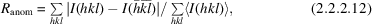where, in this case, <I(hkl)> is the mean intensity of the Friedel mates of the reflections hkl, or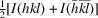. Here, the sumsrun over all unique reflections with one of the indices, typically h, greater than zero (h > 0) for which both Friedel mates have been observed at least once.

The ratio of Ranom to Rp.i.m. has been proposed as a possible indicator for the strength of the anomalous signal (Panjikar & Tucker, 2002).

Anomalous correlation coefficient, CCanom. The anomalous correlation coefficient CCanom quantifies the linear dependence of observed anomalous differences in two diffraction data sets. These can be data sets, for example, collected at two different wavelengths in a MAD experiment. In cases where only one data set is available, two randomly partitioned half data sets can be created for comparison.

Note. The correlation coefficient referred to here and elsewhere in this chapter is invariably the Pearson linear correlation coefficient (Rodgers & Nicewander, 1988):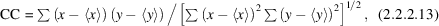with x and y being, in this case, the anomalous differences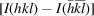or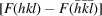in the two data sets, <x> and <y> are their averages, and the summations are over all reflections hkl for which observations exist in both data sets across the entire resolution range or within a particular resolution shell. CCanom is a reliable indicator of the strength of the anomalous signal. Values above 0.30 are considered good.

R.m.s. correlation ratio. This is another statistic based on randomly partitioned data sets, which is calculated by the program SCALA (Evans, 2006; Collaborative Computational Project, Number 4, 1994). It is an analysis of the scatterplot of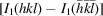versus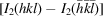, where the subscripts 1 and 2 identify the two half data sets. The analysis assumes that the correlation is ideally 1.0. The r.m.s. correlation ratio is defined as the ratio of the r.m.s. widths of the scatterplot distribution along the diagonal and perpendicular to the diagonal. This statistic seems to be more robust than CCanom to the presence of outliers. It cannot, however, be applied to analysing the correlations between different data sets.

Mean anomalous signal-to-noise ratio, <d′′/σ(d′′)>. The anomalous signal-to-noise ratio of an individual reflection measurement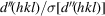is defined as the ratio of the observed anomalous intensity difference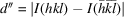and the corresponding estimated standard uncertainty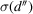in the measurement of this anomalous difference. The average of the anomalous signal-to-noise ratios for all reflections within a certain resolution range is used as an indicator of utility for phasing. A value of (2/π)1/2 ≃ 0.8 for mean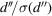of a resolution shell, for example, is taken to indicate that no anomalous signal is present (G. Sheldrick & G. Bunkoczi, personal communication).

Decay R factor, Rd. The decay R factor Rd is defined as a pairwise R factor based on the intensities of symmetry-related reflections occurring on different diffraction images (Diederichs, 2006). An increase in Rd as a function of difference in image-collection times is a good indicator of radiation damage occurring during data collection. In fractional form, this is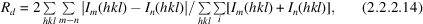where Im(hkl) and In(hkl) are the intensities of the reflection hkl occurring on images m and n. The only program in which this is currently implemented is XDSSTAT.

Wilson-plot B factor, BWilson. A Wilson plot (Wilson, 1949) is a plot for a contiguous series of resolution shells of the logarithm of the mean intensity in a given resolution shell divided by the sum of the squared atomic form factors for all atoms in the unit cell evaluated at the mean of the resolution limits of the shell. From a least-squares fit of a straight line to the linear part of the Wilson plot, the B factor BWilson can be derived. Typically, data of lower than 4.5 Å resolution are excluded from the fit. The more meaningful determinations of BWilson come from Wilson plots that are linear all the way to the nominal resolution dmin and minimize the occurrence of spikes due to ice rings.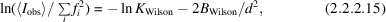where <Iobs(hkl)> is the mean over the intensities of all observed reflections hkl in a given resolution shell. The sumruns over all atoms in the structure. The parameter d is the midpoint of the resolution shell over which Iobs has been averaged. KWilson is an absolute scale factor.

### References

Arnberg, L., Hovmöller, S. & Westman, S. (1979). On the significance of `non-significant' reflexions. Acta Cryst. A35, 497–499.
Blundell, T. L. & Johnson, L. N. (1976). Protein Crystallography. New York: Academic Press.
Collaborative Computational Project, Number 4 (1994). The CCP4 suite: programs for protein crystallography. Acta Cryst. D50, 760–763.
Diederichs, K. (2006). Some aspects of quantitative analysis and correction of radiation damage. Acta Cryst. D62, 96–101.
Diederichs, K. (2010). Quantifying instrument errors in macromolecular X-ray data sets. Acta Cryst. D66, 733–740.
Diederichs, K. & Karplus, P. A. (1997a). Improved R-factors for diffraction data analysis in macromolecular crystallography. Nat. Struct. Biol. 4, 269–275.
Diederichs, K. & Karplus, P. A. (1997b). Improved R-factors for diffraction data analysis in macromolecular crystallography. Erratum. Nat. Struct. Biol. 4, 592.
Evans, P. (2006). Scaling and assessment of data quality. Acta Cryst. D62, 72–82.
Hirshfeld, F. L. & Rabinovich, D. (1973). Treating weak reflexions in least-squares calculations. Acta Cryst. A29, 510–513.
James, R. W. (1948). False detail in three-dimensional Fourier representations of crystal structures. Acta Cryst. 1, 132–134.
Kabsch, W. (1988). Evaluation of single-crystal X-ray diffraction data from a position-sensitve detector. J. Appl. Cryst. 21, 916–924.
Kabsch, W. (1993). Automatic processing of rotation diffraction data from crystals of initially unknown symmetry and cell constants. J. Appl. Cryst. 26, 795–800.
Kabsch, W. (2010). XDS. Acta Cryst. D66, 125–132.
Otwinowski, Z. & Minor, W. (1997). Processing of X-ray diffraction data collected in oscillation mode. Methods Enzymol. 276, 307–326.
Panjikar, S. & Tucker, P. A. (2002). Phasing possibilities using different wavelengths with a xenon derivative. J. Appl. Cryst. 35, 261–266.
Rodgers, J. L. & Nicewander, W. A. (1988). Thirteen ways to look at the correlation coefficient. Am. Stat. 42, 59–66.
Stenkamp, R. E. & Jensen, L. H. (1984). Resolution revisited: limit of detail in electron density maps. Acta Cryst. A40, 251–254.
Vaguine, A. A., Richelle, J. & Wodak, S. J. (1999). SFCHECK: a unified set of procedures for evaluating the quality of macromolecular structure-factor data and their agreement with the atomic model. Acta Cryst. D55, 191–205.
Weiss, M. S. (2001). Global indicators of X-ray data quality. J. Appl. Cryst. 34, 130–135.
Weiss, M. S. & Hilgenfeld, R. (1997). On the use of the merging R factor as a quality indicator for X-ray data. J. Appl. Cryst. 30, 203–205.
Wilson, A. J. C. (1949). The probability distribution of X-ray intensities. Acta Cryst. 2, 318–321.